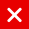# IOTA Tutorial

In this video series different topics will be explained which will help you to understand IOTA. It is recommended to watch each video sequentially as I may refer to certain IOTA topics explained earlier.

The generation of a key, digests and address, all starts with a seed.

How the key is calculated, see: https://github.com/iotaledger/iota.cr… var key = function(seed, index, length)

Every index number refers to an address, also known as public key, and each address has a corresponding unique key, also known as the private key. seed is a randomly generated 81 trytes (A-Z9), converted to trits (81×3=243 trits) index is an integer (0,1,2 .. 9007199254740991) and every address has a corresponding index number. length is the security level (1,2 or 3)

• Security level 1:
Key has 1×27 = 27 segments, each segment consists of 81 trytes. key has in total 27 x 81 = 2187 trytes
• Security level 2:
Key has 2×27 = 54 segments, each segment consists of 81 trytes. key has in total 2 x 27 x 81 = 2 x 2187 = 4374 trytes
• Security level 3:
Key has 3×27 = 81 segments, each segment consists of 81 trytes. key has in total 3 x 27 x 81 = 3 x 2187 = 6561 trytes Security level 1, key size = (2187 x 3 x ln(3) / ln(2)) / 8 = ~1300 bytes Security level 2, key size = (4374 x 3 x ln(3) / ln(2)) / 8 = ~2600 bytes Security level 3, key size = (6561 x 3 x ln(3) / ln(2)) / 8 = ~3900 bytes

How the digests is calculated, see: https://github.com/iotaledger/iota.cr… var digests = function(key)

• Security level 1, digests size = 1×243 = 243 trits = 243 / 3 = 1 x 81 trytes
• Security level 2, digests size = 2×243 = 486 trits = 486 / 3 = 2 x 81 trytes
• Security level 3, digests size = 3×243 = 729 trits = 729 / 3 = 3 x 81 trytes

• Security level 1, address = hash(digests[0-242])
• Security level 2, address = hash(digests[0-485])
• Security level 3, address = hash(digests[0-728]) Address size is always 243 trits = 243 / 3 = 81 trytes
You might also like

This website uses cookies to improve your experience. We'll assume you're ok with this, but you can opt-out if you wish. AcceptRead More

Did you know?

Buy in without the stress of trying to time the market

when you setup a savings plan. If you haven’t started a plan yet, head to your Bitpanda account to get started!

Purchasing cryptocurrencies comes with a number of risks.
Never invest money that you can't afford to lose.You can automatically invest

in IOTA with Bitpanda Savings!